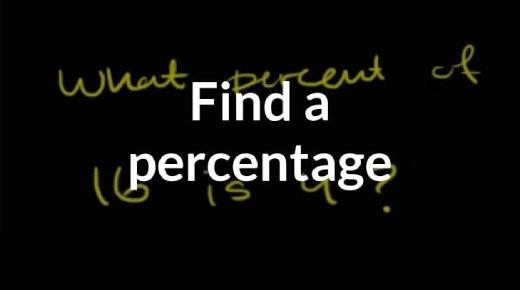# Math Trivia Quiz: Can You Pass This Percent Equations Test?

Approved & Edited by ProProfs Editorial Team
The editorial team at ProProfs Quizzes consists of a select group of subject experts, trivia writers, and quiz masters who have authored over 10,000 quizzes taken by more than 100 million users. This team includes our in-house seasoned quiz moderators and subject matter experts. Our editorial experts, spread across the world, are rigorously trained using our comprehensive guidelines to ensure that you receive the highest quality quizzes.
| By Olga Smith
O
Olga Smith
Community Contributor
Quizzes Created: 1 | Total Attempts: 135
Questions: 6 | Attempts: 135SettingsWelcome to this math trivia quiz that seeks to see if you can pass this percent equations test? In this quiz, you will be expected to come up with the number that is represented by the fraction of another number. How about you give it a try and get to see if you do indeed know all there is to percentages.

• 1.

### What number is 40% of 82.  Check all the processes  that can be used to determine the answer.

• A.

.40 multiplied by 82

• B.
• C.
• D.
A. .40 multiplied by 82
B.
C.
Explanation
To find 40% of 82, you can multiply 0.40 (which is the decimal equivalent of 40%) by 82. This will give you the answer.

Rate this question:

• 2.

### Check the following that could be applied to percent estimation for the following problem: 27% of 125

A.
B.
Explanation
Percent estimation involves approximating the value of a percentage without the need for precise calculations. In this case, to estimate 27% of 125, one could round 27% to 25% and calculate 25% of 125, which is 31.25. Since 27% is slightly higher than 25%, the estimated value would be slightly higher than 31.25. Therefore, the answer is 31.

Rate this question:

• 3.

### A cell phone survey found  75% of the age 18-25 gave up their home phone and only use a cell phone.  If 4, 264 people only use a cell phone,  about how many people were surveyed?   Determine the best option(s) you would use to determine the answer.

• A.

Percent Estimation

• B.

Percent of a number

• C.

Percent Equation

• D.

Percent Proportions

A. Percent Estimation
Explanation
The correct answer is "Percent Estimation" because in this question, we are given the percentage of people in a certain age group who gave up their home phone and only use a cell phone. We are also given the actual number of people who only use a cell phone. To determine the total number of people surveyed, we can use percent estimation by estimating the percentage of the total population represented by the given number of people who only use a cell phone.

Rate this question:

• 4.

### Using percent Proportion, check all the ways this problem can best be represented.  What number is 25% of 180?

A.
Explanation
To find what number is 25% of 180, we can set up a proportion using the percent proportion formula. The formula states that the part over the whole is equal to the percent over 100. In this case, the part is the unknown number we are trying to find, the whole is 180, and the percent is 25. Setting up the proportion, we get x/180 = 25/100. Cross-multiplying, we get 100x = 180 * 25. Solving for x, we find that x = 45. Therefore, 45 is 25% of 180.

Rate this question:

• 5.

### 40% of what number is 82?

• A.

206

• B.

210

• C.

310

• D.

205

D. 205
Explanation
To find 40% of a number, we can multiply the number by 0.4. So, if we let the number be x, we have the equation 0.4x = 82. Dividing both sides by 0.4, we find that x = 205. Therefore, 40% of 205 is 82.

Rate this question:

• 6.

### Determine what is the correct demonstration of percent equation for the following situation:  Molly had how many Legos in her box if it was 88% of 300.

A.
Explanation
Molly had 88% of 300 Legos in her box.

Rate this question:

Related TopicsBack to top